Latest Banking jobs   »   Reasoning Ability Quiz For SBI/IBPS PO...

Reasoning Ability Quiz For SBI/IBPS PO Mains 2021- 13th December

Directions (1-5): Study the following information carefully to answer the given question.
There are eight members i.e. M, N, Q, A, R, O, B and P in a family having three generation and all are related to Q in a certain way and there are only two married couple in the family. They all are sitting around a circular table such that all are facing towards the centre. Q sits third to the left of his mother-in-law, who sits second to the left of sister of R. N is unmarried. O has only one son. B is the grandson of A, who sits third to the right of N, who is the aunt of B. O is the mother of R. M is the mother-in-law of P and sits on the immediate left of brother in law of Q. N is the only daughter of M. A and O is not the married couple. Son of Q sits third to the right of Q’s brother-in-law. R is not married. N is not the immediate neighbour of P.

Q1. How is O related to Q?
(a) Mother
(b) Father
(c) Grandmother
(d) Mother-in-law
(e) None of these

Q2. Who among the following sits second to the right of son of Q?
(a) Mother of Q
(b) Father of B
(c) Father of N
(d) Brother of N
(e) None of these

Q3. How many persons sits between P and sister of Q?
(a) One
(b) Two
(c) More than Three
(d) No one
(e) Three

Q4. Who among the following is the mother of B?
(a) P
(b) M
(c) O
(d) R
(e) None of these

Q5. Who among the following sits on the immediate right of brother-in-law of Q?
(a) M
(b) Q
(c) R
(d) N
(e) O

Directions (6-8): Study the following information and answer the questions given below:
There is a figure provided in the question given below which consist of a square composed of four triangles. Some operations are performed to convert the input figure into the output figure.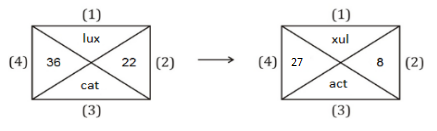For triangle 1- If the total number of letters between the first and the last letter of the word in the alphabetical series is an odd number, then all the alphabets of the word are written in reverse order.
For triangle 2- if the addition of the given two digit is less than 5 then change the number by addition of the square of its digits.
For triangle 3- If the total number of letters between the first and the last letter of the word in the alphabetical series is an even number, then the first and second letter of the word are interchange with each other.
For triangle 4-if the difference between given two digits is more than 2 then change the number by the difference of the square of its digits
Based on the above example, find the output figure of the following input.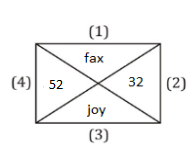Q6. How many meaningful words of six letter words can be formed by combining the letters of the output figure?
(a) Four
(b) Two
(c) Three
(d) One
(e) None

Q7. What is the difference between the digits of the triangle -2 and triangle-4 of the output figure?
(a) 21
(b) 11
(c) 32
(d) 48
(e) None of these

Q8. Which of the following letter represents the element of triangle 1 of the output figure?
(a) xaf
(b) afx
(c) ojy
(d) joy
(e) Both(a) and(c)

Directions (9-11): Each of the questions below consists of a question and two statements numbered I and II given below it. You have to decide whether the data provided in the statement are sufficient to answer the question. Read both the statements and Give answer:

Q9. Mr. X prepared different food item on the different days of the week starting from Monday to Friday, then which of the following food item is prepared on Thursday?
I. Only one food is prepared between Dhosa and Pasta, which is prepared on Wednesday. Chicken prepared before biryani.
II. Dhosa is prepared on the day after Biryani. There are two food items prepared between Biryani and Chicken. Dhokla is prepare before Biryani.

(a) If the data in statement I alone are sufficient to answer the question, while the data in statement II alone are not sufficient to answer the question.
(b) If the data in statement II alone are sufficient to answer the question, while the data in statement I alone are not sufficient to answer the question.
(c) If the data either in statement I alone or in statement II alone are sufficient to answer the question.
(d) If the data even in both statements I and II together are not sufficient to answer the question.
(e) If the data in both statements I and II together are necessary to answer the question.

Q10. What is the position of Romil with respect to jugail?
I. Romil’s rank is 27th from the left and Jugail rank is 21st from the right in a class of 40 student.
II. Rani who is on the immediate left of Romil in a class of 45 students. Rani rank is 27th from the left end. Jugail is 22nd rank from the right.

(a) If the data in statement I alone are sufficient to answer the question, while the data in statement II alone are not sufficient to answer the question.
(b) If the data in statement II alone are sufficient to answer the question, while the data in statement I alone are not sufficient to answer the question.
(c) If the data either in statement I alone or in statement II alone are sufficient to answer the question.
(d) If the data even in both statements I and II together are not sufficient to answer the question.
(e) If the data in both statements I and II together are necessary to answer the question.

Q11. How G is related to K?
I. A is the brother of K, who is married to N, who is mother of H who is married to M. M is the father of G.
II. G is the brother of R. K is the sister of N. A is married to K. J is only child of A and is married to G.

(a) If the data in statement I alone are sufficient to answer the question, while the data in statement II alone are not sufficient to answer the question.
(b) If the data in statement II alone are sufficient to answer the question, while the data in statement I alone are not sufficient to answer the question.
(c) If the data either in statement I alone or in statement II alone are sufficient to answer the question.
(d) If the data even in both statements I and II together are not sufficient to answer the question.
(e) If the data in both statements I and II together are necessary to answer the question.

Directions (12-14): Study the following information and answer the questions given below:
There are AB axis in such a way that A is in north and B is in south direction. There is XY axis in such a way that X is in west direction and Y is in east direction. AB axis and XY axis intersect at a point Q in such a way that AQ is 15m, QB is 17m, QX is 12m, QY is 24 m.
Mr. Z starts walking along the QX line towards Q from X and after walking 4m he takes a left turn and walks for 6m to reach point S. Mr. L starts walking in the south direction from Y and walks 4m to stop at point K. Mr. D walks towards Q from A and walks for 8m and takes a left turn and walks for 3m to reach point I. Mr. M starts walking in the north direction from point B and walks for 13m and then takes a right turn and walks for 3m to reach point N.

Q12.What is the shortest distance between point S and point N?
(a) √231 m
(b) √221 m
(c) √211 m
(d) 13 m
(e) None of these

Q13. How far and in which direction is point K with respect to point N?
(a) 21m west
(b) 24m west
(c) 22m west
(d) 21m east
(e) 22m east

Q14. In which direction is point S with respect to Mr. M’s final position?
(a) Southeast
(b) North
(c) Northeast
(d) Southwest
(e) Northwest

Q15. In the following question a statement is given followed by two more statements numbered as I, II and III. You have to read both the statement and decide which of the given assumptions follows the statement.
Statement: “SSC aspirants are fade up with Paper leak and most of them have decided to start preparing for banking field.”- An article published in The Hindu.
Assumption:
(I) SSC has not taken any corrective measures to stop paper leak.
(II) There will be no paper leak in case of banking examination.
(III) There is a great future in banking field.
(a) Only II follows
(b) Both I and III follow
(c) Only III follows
(d) Both I and II follow
(e) None follows

Solutions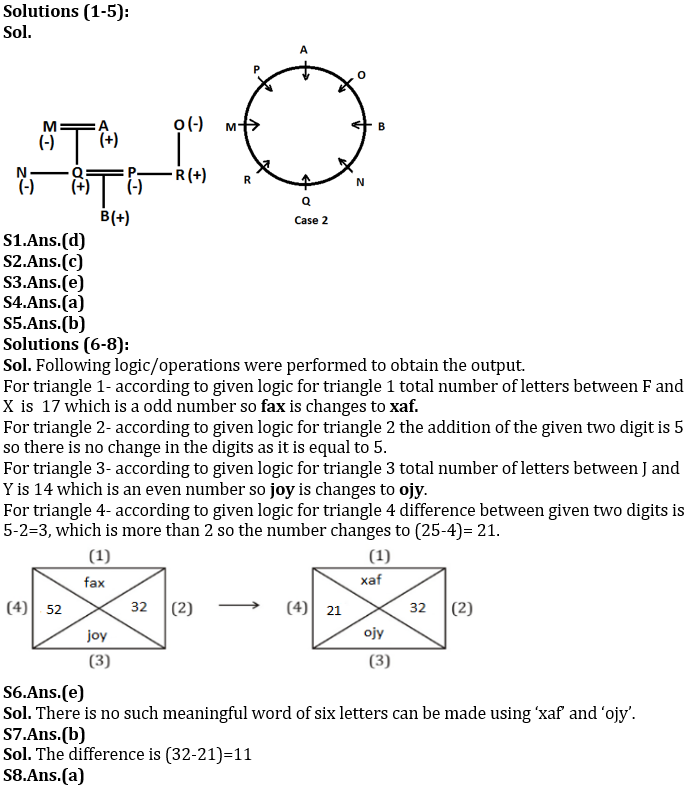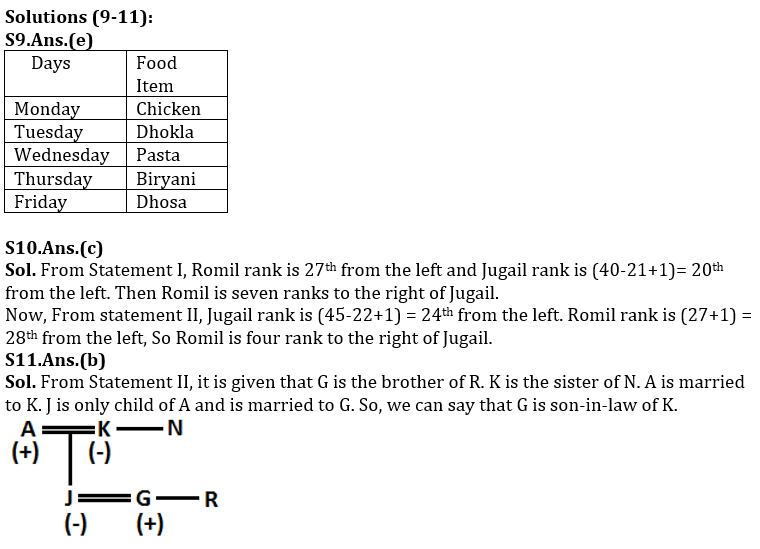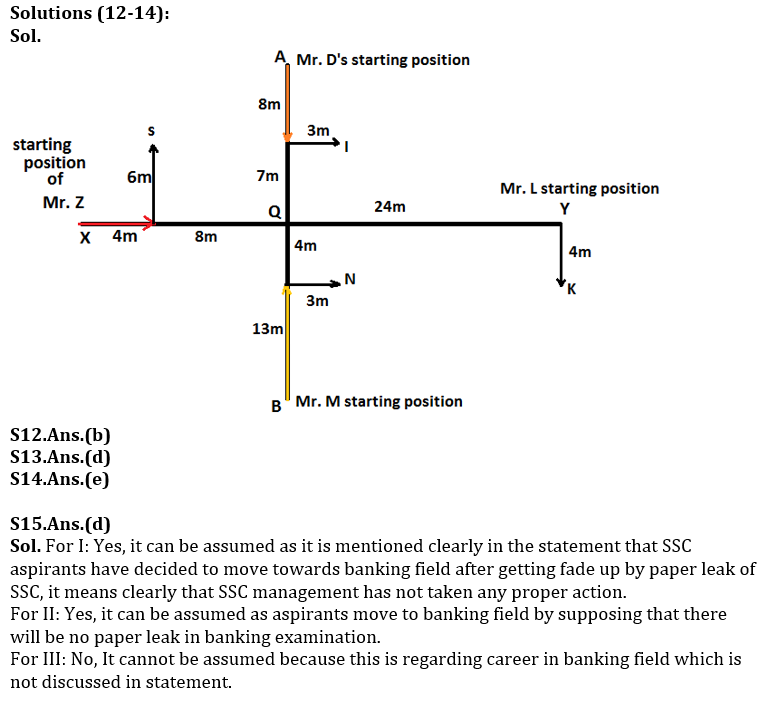×

Thank You, Your details have been submitted we will get back to you.Join India's largest learning destination

What You Will get ?

•Job Alerts
•Daily Quizzes
•Subject-Wise Quizzes
•Current Affairs
•Previous year question papers
•Doubt Solving session

ORJoin India's largest learning destination

What You Will get ?

•Job Alerts
•Daily Quizzes
•Subject-Wise Quizzes
•Current Affairs
•Previous year question papers
•Doubt Solving session

ORJoin India's largest learning destination

What You Will get ?

•Job Alerts
•Daily Quizzes
•Subject-Wise Quizzes
•Current Affairs
•Previous year question papers
•Doubt Solving session

Enter the email address associated with your account, and we'll email you an OTP to verify it's you.Join India's largest learning destination

What You Will get ?

•Job Alerts
•Daily Quizzes
•Subject-Wise Quizzes
•Current Affairs
•Previous year question papers
•Doubt Solving session

Enter OTP

Please enter the OTP sent to
/6

Did not recive OTP?

Resend in 60sJoin India's largest learning destination

What You Will get ?

•Job Alerts
•Daily Quizzes
•Subject-Wise Quizzes
•Current Affairs
•Previous year question papers
•Doubt Solving sessionJoin India's largest learning destination

What You Will get ?

•Job Alerts
•Daily Quizzes
•Subject-Wise Quizzes
•Current Affairs
•Previous year question papers
•Doubt Solving session

Almost there

+91Join India's largest learning destination

What You Will get ?

•Job Alerts
•Daily Quizzes
•Subject-Wise Quizzes
•Current Affairs
•Previous year question papers
•Doubt Solving session

Enter OTP

Please enter the OTP sent to Edit Number

Did not recive OTP?

Resend 60

By skipping this step you will not recieve any free content avalaible on adda247, also you will miss onto notification and job alerts

Are you sure you want to skip this step?

By skipping this step you will not recieve any free content avalaible on adda247, also you will miss onto notification and job alerts

Are you sure you want to skip this step?